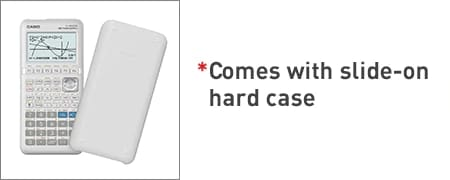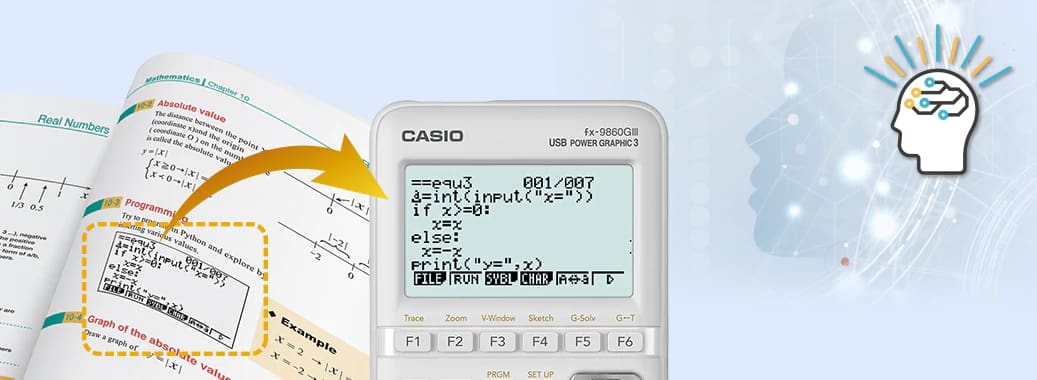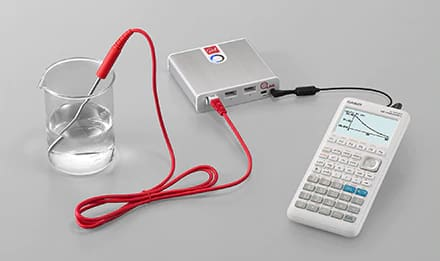# fx-9860GIII | CASIO

Search

Python
Graphing Calculators

# fx-9860GIII

My GShock

## Features## User-friendly Interface

• Large display (128 × 64 dots)
•  High-resolution LCD
• High-speed CPU
• Rectangular coordinate graphing, Polar coordinate graphing
• Parametric function graphing, Inequality graphing
• Table and Graph
• Dual graph (table and graph, graph and graph)
• Solve (root, minimum, maximum, intersection, integration)
• Dynamic graph
• Conic section graph
• Recursion graph
• eActivity
• Geometry
• Statistical plot (scatter plot, xyLine, normal probability plot, histogram, box plot)
• Statistical regression graphs (linear, med-med, quadratic, cubic, quartic, logarithmic, exponential, power, sinusoidal, logistic regression)
• Advanced statistical calculations: tests, intervals, distributions
• Pie chart
• Bar graph
• Numeric equation solver, Simultaneous equations, Polynomial equations
• Financial functions
• Programming
• Examination Mode
• Python
• Catalog Function
• Data communication
• Out-of-the-box USB operations
• Direct connection to a projector
• User memory: 62,000 bytes, User storage memory: 3 M bytes

## Programming language “Python”

The fx-9860GIII comes built-in with Python, a programming language used in the development of Internet search engines, social media sites, robots, etc.### Learning algorithmic thinking with Python*

Python is attracting attention in the field of education as a learning tool that cultivates algorithmic thinking and has been adopted for use in textbooks.

##### Python mode supports a version of MicroPython Version 1.9.4, which has been adapted to run on this calculator. Please note that MicroPython is different from the Python that runs on a computer.## Communicate with PC easily without software

By connecting fx-9860GIII to a PC with a USB cable, you can easily communicate with PC. It is convenient because data such as programs and memory can be easily moved between fx-9860GIII and PC.##### Communicate between fx-9860III and PC with USB cable